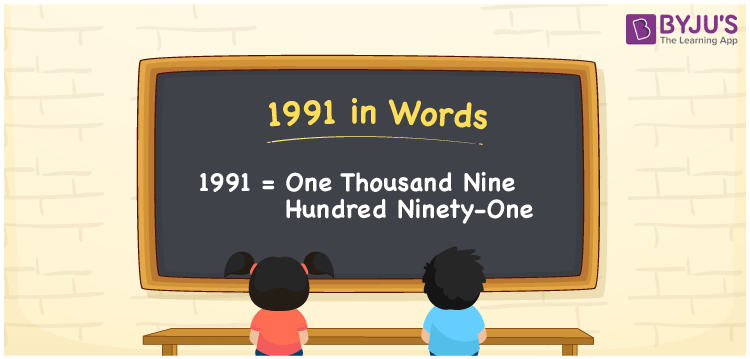# 1991 in Words

1991 in words can be written as One Thousand Nine Hundred Ninety-One. The units, tens, hundreds and thousands places of the number 1991 are made use of to write the number name. If you buy a leather jacket for Rs. 1991, then you can say that “I bought a leather jacket for One Thousand Nine Hundred Ninety-One”. In this article, you will learn the spelling of  numbers in words with the help of the English alphabet. Hence, the number 1991 can be read as “One Thousand Nine Hundred Ninety-One” in words.

 1991 in words One Thousand Nine Hundred Ninety-One One Thousand Nine Hundred Ninety-One in Numbers 1991

## 1991 in English Words## How to Write 1991 in Words?

To write the number 1991 in English, we have to first find the place value of each digit. The place value chart of 1991 is given below.

 Thousands Hundreds Tens Ones 1 9 9 1

The expanded form of 1991 is provided below:

1 x Thousand + 9 x Hundred + 9 × Ten + 1 × One

= 1 x 1000 + 9 x 100 + 9 x 10 + 1 x 1

= 1000 + 900 + 90 + 1

= 1991

= One Thousand Nine Hundred Ninety-One

Therefore, 1991 in words is written as One Thousand Nine Hundred Ninety-One.

1991 is a natural number that precedes 1992 and succeeds 1990.

1991 in words – One Thousand Nine Hundred Ninety-One

Is 1991 an odd number? – Yes

Is 1991 an even number? – No

Is 1991 a perfect square number? – No

Is 1991 a perfect cube number? – No

Is 1991 a prime number? – No

Is 1991 a composite number? – Yes

## Frequently Asked Questions on 1991 in Words

Q1

### How do you write the number 1991 in words?

1991 in words is One Thousand Nine Hundred Ninety-One.
Q2

### Find the value of 2000 minus 9.

The value of 2000 minus 9 is 1991. Therefore, 1991 in words is One Thousand Nine Hundred Ninety-One.
Q3

### Is 1991 a perfect square?

No, 1991 is not a perfect square as it cannot be expressed as the product of two integers which are equal.